#### You may also like### Days and Dates

Investigate how you can work out what day of the week your birthday will be on next year, and the year after...### Prime Magic

Place the numbers 1, 2, 3,..., 9 one on each square of a 3 by 3 grid so that all the rows and columns add up to a prime number. How many different solutions can you find?### Is it Magic or Is it Maths?

Here are three 'tricks' to amaze your friends. But the really clever trick is explaining to them why these 'tricks' are maths not magic. Like all good magicians, you should practice by trying them. Can you explain how they work?

# Overlaps

##### Age 11 to 14Challenge Level
Seven Regions

Holly from Malet Lamber School managed to find the following way of arranging the numbers $1$ to $7$, with the numbers in each circle adding up to $13$: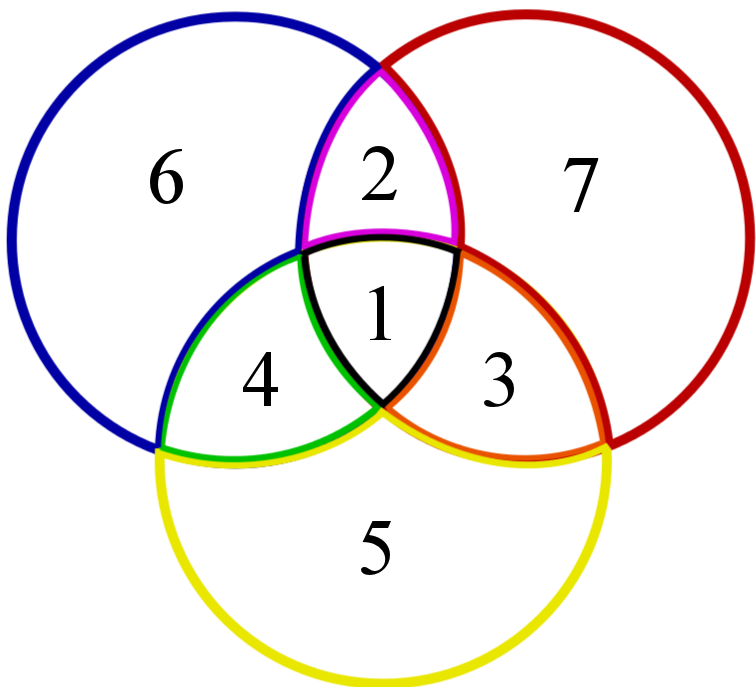Michael from Sevenoaks found a different way of arranging the numbers to give a total of $13$ in each circle: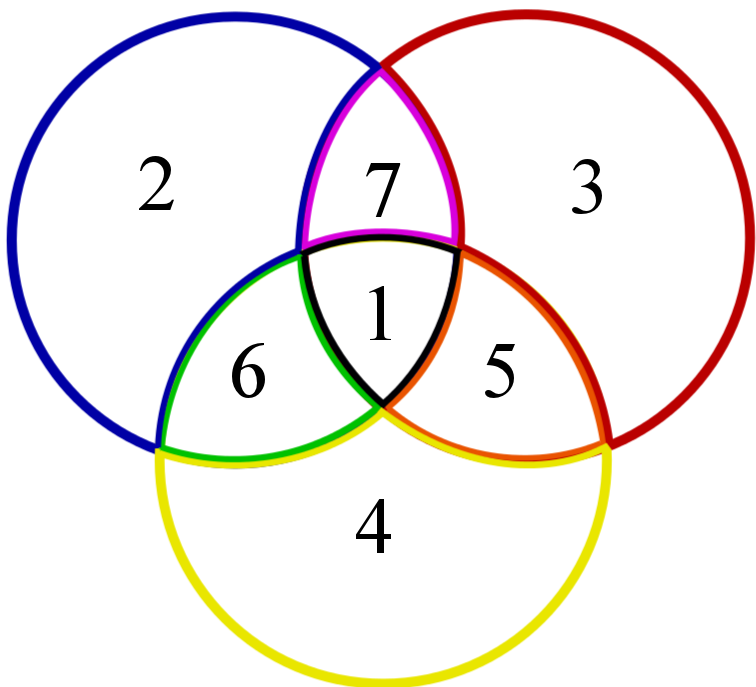Jack from Hitchin Boys' School found an arrangement where the numbers in each circle added to $14$: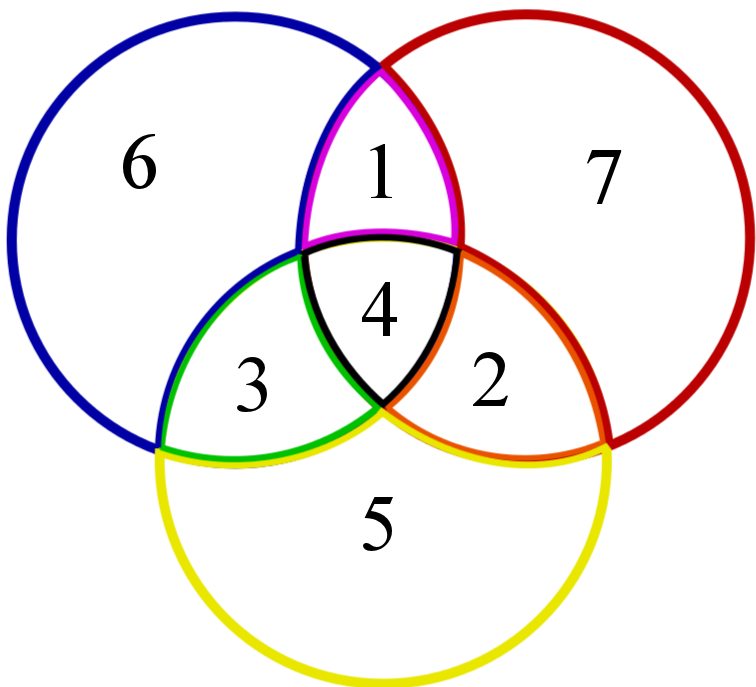Hazel from Kings Norton Girls' School found these and two more solutions - each had $7$ in the central area, but in one solution the numbers in all the circles totalled $16$ and in the other they totalled $19$: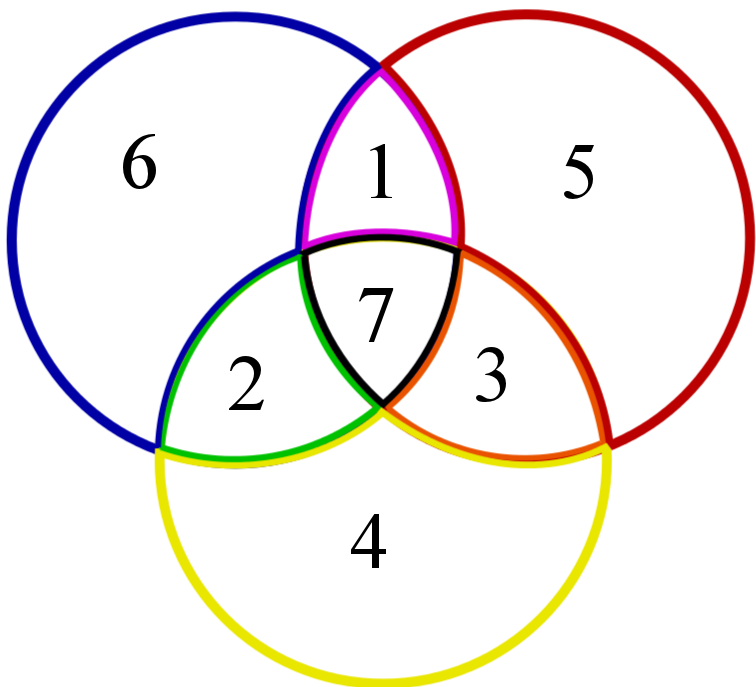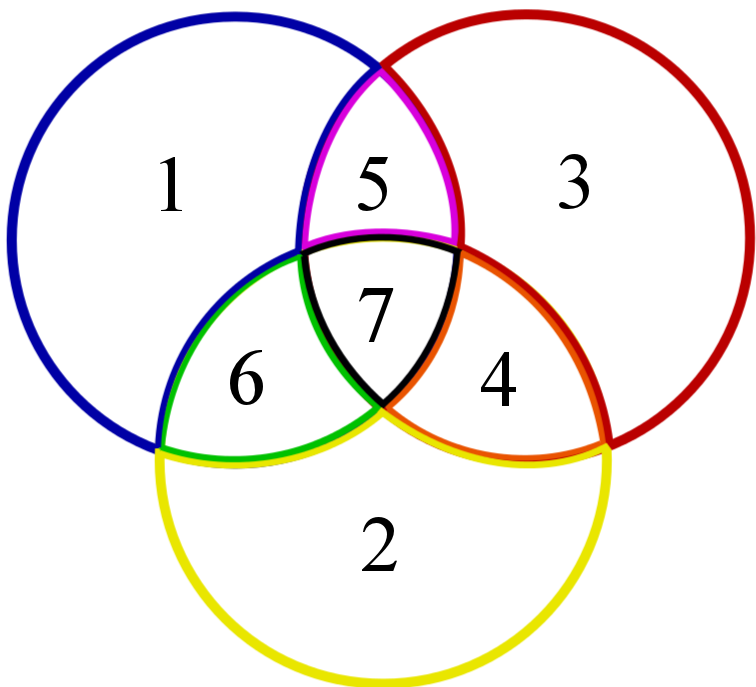Hazel says:

I tried other combinations but was not successful. I did try for the missing numbers between $13$ and $19$ (my lowest/ highest solutions) but was
not able to find answers for $15$, $17$, or $18$.

Julian from British School Manila in the Philippines explains why it is impossible to have each circle totalling $16$ with $4$ in the centre:

If $4$ is in the centre, this means each circle contains $4$ numbers, one of which is $4$, which add to $16$. This means we need to find combinations of three of the numbers $1$, $2$, $3$, $5$, $6$, $7$ which add up to $12$. But we can only find two combinations which work: $1,5,6$ and $2,3,7$ and three circles, so we can't find a valid solution.

Pablo, Patricia, Javier and Sara from Kings College Alicante in Spain explained why our total must always be between $13$ and $19$:

The number you put in the centre will be counted $3$ times. The three numbers you put around the centre will each be counted twice.

To get a total of $13$, you must have $1$ in the centre because if
you put the smallest number, $1$, the total must be the smallest because the
centre is counted three times. Around the centre you put the next smallest
numbers because they are counted twice, and then the largest in the outer
space.

To show $19$ is the biggest possible total, we put the biggest number, $7$, in the
centre because it is counted three times, then the next largest numbers around
the centre, as they are counted twice. Then we put the smallest numbers in the
outer space, as they are counted once. This gave us the biggest total we could have, which turned out to be $19$.

Thank you to everyone who submitted a solution to this problem!

Five Rings

Sean from Sacred Heart Catholic College found a solution here where the total of each ring is $11$.

Nabihah from Paddington Academy found a solution where the total of each ring is $13$:

According to the picture on the website:
$a=9$
$b=4$
$c=1$
$d=8$
$e=3$
$f=2$
$g=5$
$h=6$
$i=7$.

I figured out that the total had to be between $10$ and $15$ so i tried $13$ and
created the only possibilities that could add up to $13$, which were $9$ and $4$,
$6$ and $7$, $8$ and $5$. I tried with many different combinations using the
possibilities and I eventually solved the problem.

Eva from King ´s College, The British School of Alicante, found a solution where the total of each ring is $14$:

I have found a solution for the "Five Rings" Problem for which
all of the circles sum to $14$. I tried other circle sums such as $10$, $11$ and
$12$ but I did not find solutions for these. My method was simply to
experiment with many different combinations.

My solution reads (from left to right); $8,6,1,7,4,3,2,9,5$.

Zach found each of these solutions, and explains clearly why no other totals can be made here.